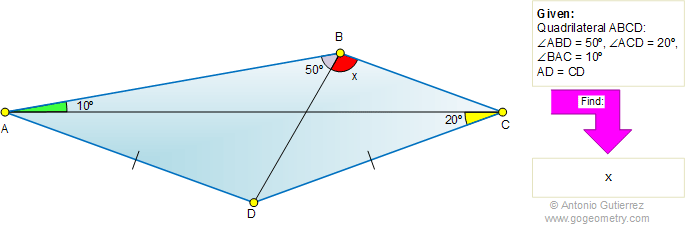# Online Geometry Problem 425: Quadrilateral, Triangle, Angles, 10, 20, 50 degrees, Congruence

 The figure shows a quadrilateral ABCD with angle ABD = 50 degrees, angle ACD = 20 degrees, angle BAC = 10 degrees, and AD = CD. Find the measure of angle CBD.Home | Search | Geometry | Problems | All Problems | 421-430 | Email | Solution / comment | By Antonio Gutierrez